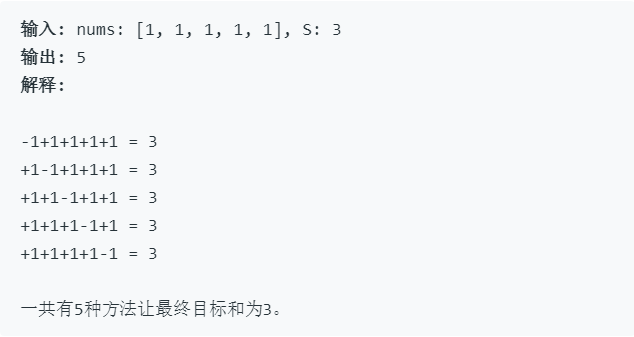# leetcode 494——目标和1. 数组非空，且长度不会超过20。
2. 初始的数组的和不会超过1000。
3. 保证返回的最终结果能被32位整数存下。

• 原问题等同于： 找到nums一个正子集和一个负子集，使得总和等于target
• 我们假设P是正子集，N是负子集 例如： 假设nums = [1, 2, 3, 4, 5]，target = 3，一个可能的解决方案是+1-2+3-4+5 = 3 这里正子集P = [1, 3, 5]和负子集N = [2, 4]
• 那么让我们看看如何将其转换为子集求和问题：
sum( P) - sum(N) = target
sum( P) + sum(N) + sum( P) - sum(N) = target + sum( P) + sum(N)
2 * sum( P) = target + sum(nums)

class Solution {
public:
int findTargetSumWays(vector<int>& nums, int S) {
if (nums.empty())
return 0;

int sum = 0;
for (auto i : nums) {
sum += i;
}

if (sum < S || (sum + S) % 2 == 1)
return 0;

int sumP = (sum + S) >> 1;

//因此转化为求nums中和为 sumP 的子数组的个数
return sumsetSum(nums, sumP);
}

private:
int sumsetSum(const vector<int>& nums, int s) {
vector<int> dp(s+1);    //dp[i]表示和为i的子数组的个数
dp = 1;

for (int n : nums) {
for (int curSum = s; curSum >= n; --curSum) {
dp[curSum] += dp[curSum-n];
}
}

return dp[s];
}
};

01-3082
12-313008-20175
03-3128
09-05122
02-2954
02-17111
11-18839
11-28262